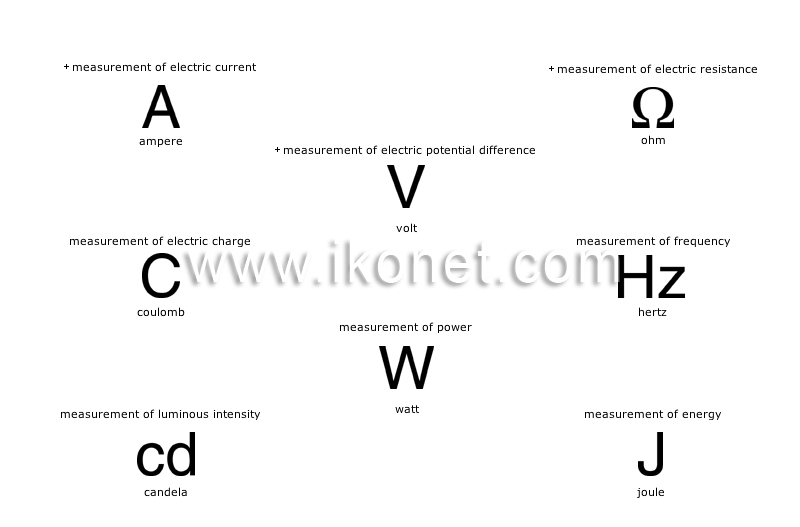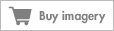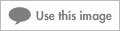Look up an image:

#### international system of unitsDecimal system established by the 11th General Conference on Weights and Measures (GCWM) in 1960 and used by many countries.See international system of units in : french | spanish

#### voltDifference in potential between two points of a conductor carrying a constant current of 1 ampere when the power between these points is 1 watt.

#### ohmElectrical resistance between two points of a conductor carrying a current of 1 ampere when the difference in potential between them is 1 volt.

#### candelaUnit of light intensity equivalent to a radiant intensity of 1/683 watts per steradian (solid angle).

#### coulombAmount of electricity carried in 1 second by a current of 1 ampere.

#### jouleAmount of energy released by the force of 1 newton acting through a distance of 1 meter.

#### wattEnergy transfer of 1 joule during 1 second.

#### hertzFrequency of a periodic phenomenon whose period is 1 second.

#### ampereConstant current of 1 joule per second in a conductor.